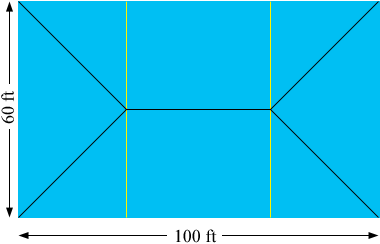SEARCH HOMEMath Central Quandaries & QueriesQuestion from Jody: I am digging a pond that is 100' X 60' X 10' deep in the centre. All four sides will have 3-1 slopes on them. How do I calculate how may yards of material will have to be removed?Hello Jody,
I will try to help you get started.  I assume that “3-1 slopes” means that there is a 1 foot drop in soil for every 3 feet of distance (it makes the numbers nicer, but it could also be the other way [the diagram will change if so]).  Let’s take a look at a bird’s eye picture:This picture is to scale, so this is how it would actually look.
Imagine making cuts through the yellow lines and gluing the two end pieces together.  We would have two new shapes:  a right triangular prism and a square based pyramid.
What are the formulae for the volumes of these shapes?  What is the total volume?
Write back if you need more help.
TylerMath Central is supported by the University of Regina and The Pacific Institute for the Mathematical Sciences.